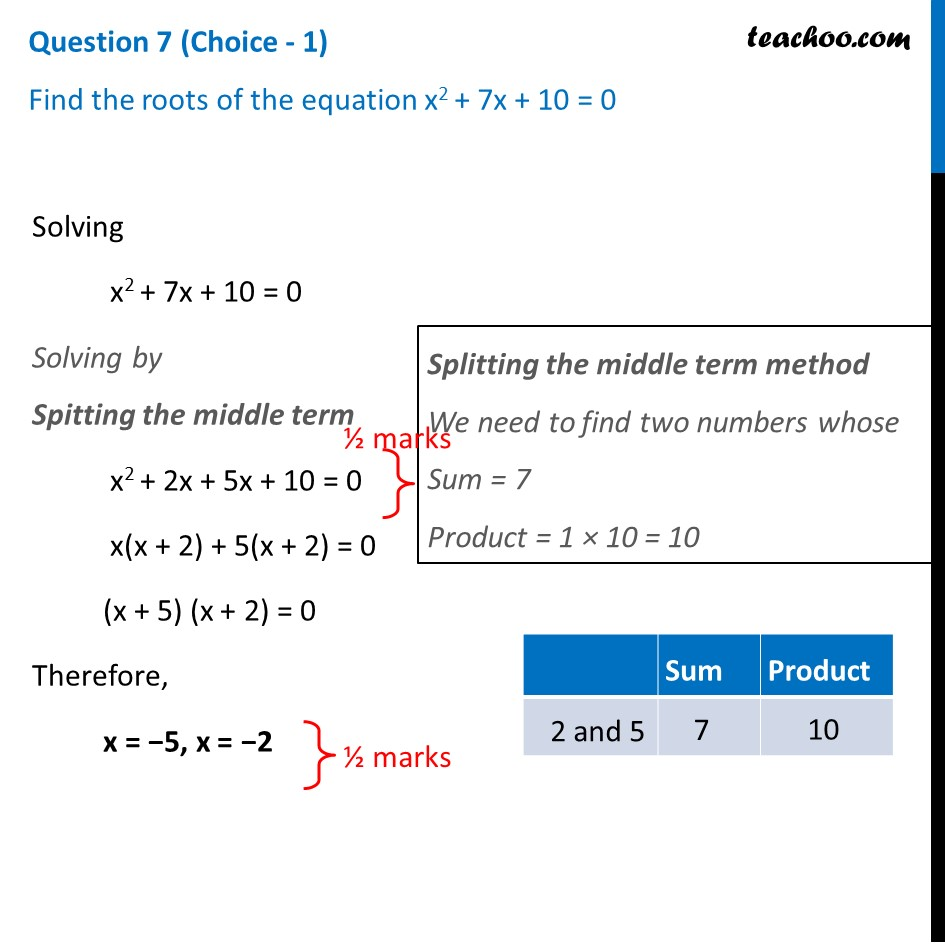## Find the roots of the equation x 2 + 7x + 10 = 01. Class 10
2. Solutions of Sample Papers for Class 10 Boards
3. CBSE Class 10 Sample Paper for 2021 Boards - Maths Standard

Transcript

Question 7 (Choice - 1) Find the roots of the equation x2 + 7x + 10 = 0 Solving x2 + 7x + 10 = 0 Solving by Spitting the middle term Splitting the middle term method We need to find two numbers whose Sum = 7 Product = 1 × 10 = 10 x2 + 2x + 5x + 10 = 0 x(x + 2) + 5(x + 2) = 0 (x + 5) (x + 2) = 0 Therefore, x = −5, x = −2

CBSE Class 10 Sample Paper for 2021 Boards - Maths Standard

Class 10
Solutions of Sample Papers for Class 10 Boards